# Wilcox Solar Observatory Magnetograms

## #8230 - 8239, 2005.03.16 - 2005.04.06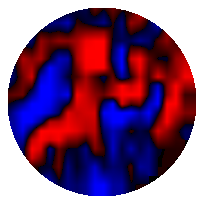```WSO Magnetogram sn=8230	 observed at 2005:03:16_21h:21m:12s
Central Meridian Carrington Longitude CT2027:108
Sky = 3 (5 worst)
trouble=00004000 NOTE:
12 intensity variance exceeds 5.0
```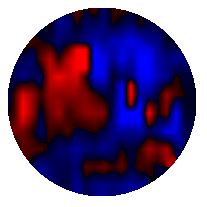```WSO Magnetogram sn=8231	 observed at 2005:03:25_19h:33m:34s
Central Meridian Carrington Longitude CT2028:350
Sky = 1 (5 worst)
trouble=00004000 NOTE:
12 intensity variance exceeds 5.0
``````WSO Magnetogram sn=8232	 observed at 2005:03:28_16h:57m:28s
Central Meridian Carrington Longitude CT2028:312
Sky = 1 (5 worst)
trouble=00004000 NOTE:
12 intensity variance exceeds 5.0
```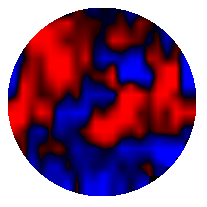```WSO Magnetogram sn=8233	 observed at 2005:03:30_21h:21m:43s
Central Meridian Carrington Longitude CT2028:283
Sky = 0 (5 worst)
trouble=00004000 NOTE:
12 intensity variance exceeds 5.0
```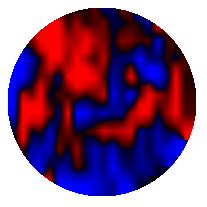```WSO Magnetogram sn=8234	 observed at 2005:03:31_21h:34m:10s
Central Meridian Carrington Longitude CT2028:270
Sky = 1 (5 worst)
trouble=00004000 NOTE:
12 intensity variance exceeds 5.0
``````WSO Magnetogram sn=8235	 observed at 2005:03:31_23h:45m:06s
Central Meridian Carrington Longitude CT2028:268
Sky = 1 (5 worst)
trouble=00004000 NOTE:
12 intensity variance exceeds 5.0
```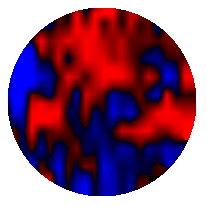```WSO Magnetogram sn=8236	 observed at 2005:04:01_22h:20m:15s
Central Meridian Carrington Longitude CT2028:256
Sky = 3 (5 worst)
trouble=00004000 NOTE:
12 intensity variance exceeds 5.0
```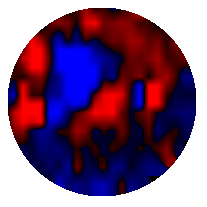```WSO Magnetogram sn=8237	 observed at 2005:04:04_23h:13m:33s
Central Meridian Carrington Longitude CT2028:216
Sky = 2 (5 worst)
trouble=00004000 NOTE:
12 intensity variance exceeds 5.0
```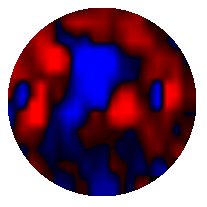```WSO Magnetogram sn=8238	 observed at 2005:04:05_21h:43m:00s
Central Meridian Carrington Longitude CT2028:204
Sky = 0 (5 worst)
trouble=00004000 NOTE:
12 intensity variance exceeds 5.0
```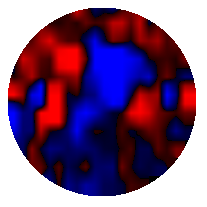```WSO Magnetogram sn=8239	 observed at 2005:04:06_18h:54m:23s
Central Meridian Carrington Longitude CT2028:192
Sky = 3 (5 worst)
trouble=00004000 NOTE:
12 intensity variance exceeds 5.0
```